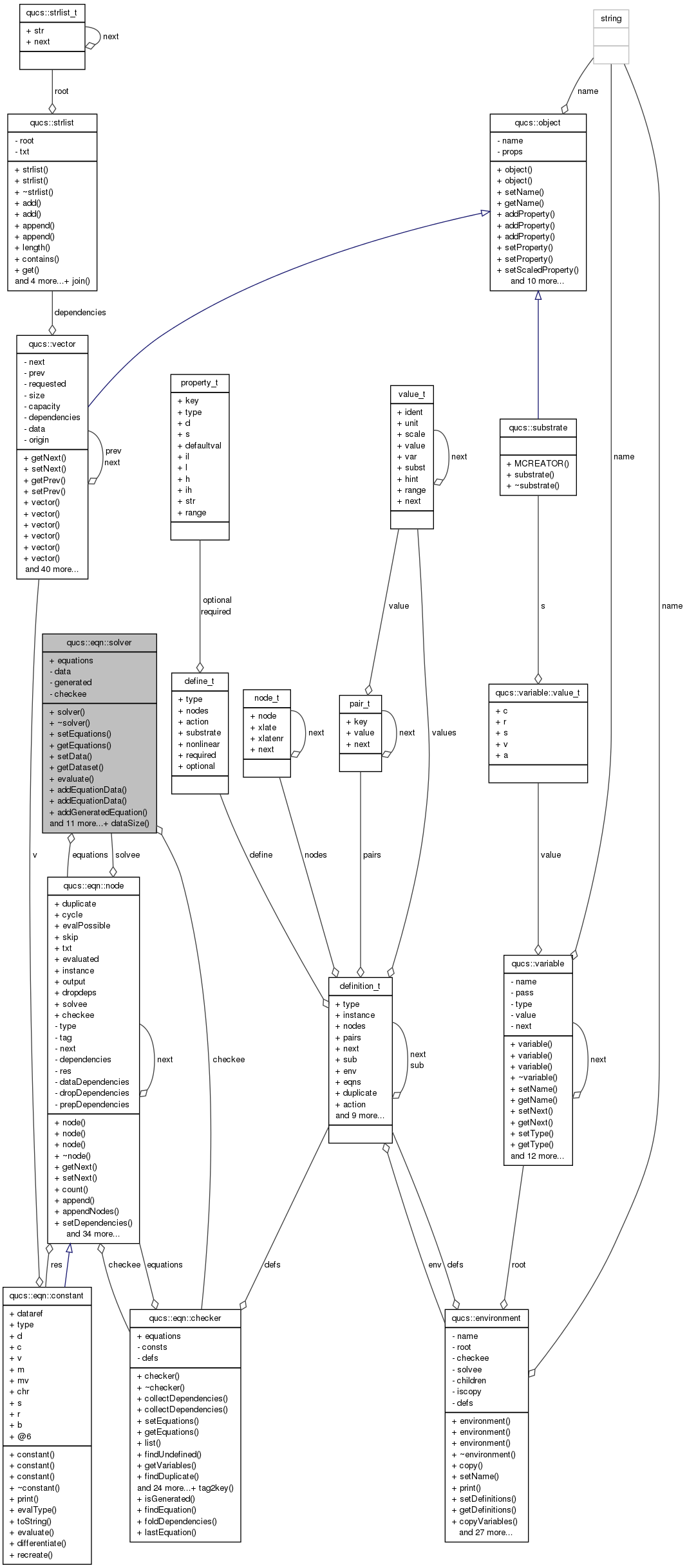Qucs-core  0.0.19
qucs::eqn::solver Class Reference

`#include <equation.h>`

Collaboration diagram for qucs::eqn::solver:[legend]

## Public Member Functions

solver (checker *)
~solver ()
void setEquations (node *eqn)
nodegetEquations (void)
void setData (dataset *d)
dataset * getDataset (void)
void evaluate (void)
nodeaddEquationData (qucs::vector *, bool ref=false)
nodeaddEquationData (matvec *)
nodeaddGeneratedEquation (qucs::vector *, const char *)
qucs::vector * dataVector (node *)
void checkinDataset (void)
void checkoutDataset (void)
int getDependencySize (strlist *, int)
int getDataSize (char *)
strlist * collectDataDependencies (node *)
int dataSize (strlist *)
qucs::vector * getDataVector (char *)
void findMatrixVectors (qucs::vector *)
char * isMatrixVector (char *, int &, int &)
int findEquationResult (node *)
int solve (dataset *)

## Static Public Member Functions

static int dataSize (constant *)

nodeequations

dataset * data
int generated
checkercheckee

## Detailed Description

Definition at line 324 of file equation.h.

## Constructor & Destructor Documentation

 qucs::solver::solver ( checker * c )

Definition at line 1872 of file equation.cpp.

 qucs::solver::~solver ( )

Definition at line 1881 of file equation.cpp.

## Member Function Documentation

 node * qucs::solver::addEquationData ( qucs::vector * v, bool ref = `false` )

Definition at line 1930 of file equation.cpp.

 node * qucs::solver::addEquationData ( matvec * mv )

Definition at line 2165 of file equation.cpp.

 node * qucs::solver::addGeneratedEquation ( qucs::vector * v, const char * n )

Definition at line 1946 of file equation.cpp.

 void qucs::solver::checkinDataset ( void )

Definition at line 2037 of file equation.cpp.

 void qucs::solver::checkoutDataset ( void )

Definition at line 2324 of file equation.cpp.

 strlist * qucs::solver::collectDataDependencies ( node * eqn )

Definition at line 2265 of file equation.cpp.

 int qucs::solver::dataSize ( constant * eqn ) ` [static]`

Definition at line 2180 of file equation.cpp.

 int qucs::solver::dataSize ( strlist * deps )

Definition at line 2222 of file equation.cpp.

 qucs::vector * qucs::solver::dataVector ( node * eqn )

Definition at line 1969 of file equation.cpp.

 void qucs::solver::evaluate ( void )

Definition at line 1892 of file equation.cpp.

 int qucs::solver::findEquationResult ( node * eqn )

Definition at line 2381 of file equation.cpp.

 void qucs::solver::findMatrixVectors ( qucs::vector * v )

Definition at line 2068 of file equation.cpp.

 dataset* qucs::eqn::solver::getDataset ( void ) ` [inline]`

Definition at line 332 of file equation.h.

 int qucs::solver::getDataSize ( char * var )

Definition at line 2199 of file equation.cpp.

 qucs::vector * qucs::solver::getDataVector ( char * str )

Definition at line 2238 of file equation.cpp.

 int qucs::solver::getDependencySize ( strlist * deps, int idx )

Definition at line 2208 of file equation.cpp.

 node* qucs::eqn::solver::getEquations ( void ) ` [inline]`

Definition at line 330 of file equation.h.

 char* qucs::eqn::solver::isMatrixVector ( char * , int & , int & )
 void qucs::eqn::solver::setData ( dataset * d ) ` [inline]`

Definition at line 331 of file equation.h.

 void qucs::eqn::solver::setEquations ( node * eqn ) ` [inline]`

Definition at line 329 of file equation.h.

 int qucs::solver::solve ( dataset * data )

Definition at line 2410 of file equation.cpp.

## Field Documentation

 checker* qucs::eqn::solver::checkee` [private]`

Definition at line 357 of file equation.h.

 dataset* qucs::eqn::solver::data` [private]`

Definition at line 355 of file equation.h.

Definition at line 352 of file equation.h.

 int qucs::eqn::solver::generated` [private]`

Definition at line 356 of file equation.h.

The documentation for this class was generated from the following files: